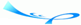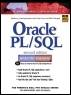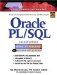# Chapter 10 Exceptions

 Team-FlyOracle® PL/SQL® Interactive Workbook, Second EditionBy Benjamin Rosenzweig, Elena Silvestrova Table of Contents Appendix D.  Answers to Test Your Thinking Sections

 1) Create the following script. For each section determine the number of students registered. If this number is equal to or greater than 15, raise the user-defined exception e_too_many_students and display the error message. Otherwise, display how many students are in a section. Make sure that your program is able to process all sections. A1: Answer: Your answer should look similar to the following:` SET SERVEROUTPUT ON SIZE 5000 DECLARE CURSOR section_cur IS SELECT section_id FROM section; v_total NUMBER; e_too_many_students EXCEPTION; BEGIN FOR section_rec in section_cur LOOP BEGIN -- calculate number of students enrolled SELECT COUNT(*) INTO v_total FROM enrollment WHERE section_id = section_rec.section_id; IF v_total >= 15 THEN RAISE e_too_many_students; ELSE DBMS_OUTPUT.PUT_LINE ('There are '||v_total|| ' students for section ID '|| section_rec.section_id); END IF; EXCEPTION WHEN e_too_many_students THEN DBMS_OUTPUT.PUT_LINE ('There are too many '|| students for '||section_rec.section_id); END; END LOOP; END; `In this script, you declare a cursor on the SECTION table. Next, for each section ID returned by the cursor, the number of students enrolled in a given section is computed. If this number equals to or greater than 15, the user-defined exception E_TOO_MANY_STUDENTS is raised. Otherwise, the message specifying how many students are enrolled in a given section is displayed.When run, this exercise produces the following output (due to the size of the output, only a part of it is shown here):` There are 0 students for section ID 79 There are 1 students for section ID 80 There are 3 students for section ID 81 There are 2 students for section ID 82 There are 2 students for section ID 83 There are 2 students for section ID 84 There are 5 students for section ID 85 There are 6 students for section ID 86 There are 7 students for section ID 87 There are 5 students for section ID 88 There are 12 students for section ID 89 … There are 5 students for section ID 155 There are 8 students for section ID 156 PL/SQL procedure successfully completed. ` 2) Modify the script you created in the previous exercise. Once the exception e_too_many_students has been raised in the inner block, re-raise it in the outer block. A2: Answer: Your answer should look similar to the following. All changes are shown in bold letters.` SET SERVEROUTPUT ON SIZE 5000 DECLARE CURSOR section_cur IS SELECT section_id FROM section; v_total NUMBER; e_too_many_students EXCEPTION; BEGIN FOR section_rec in section_cur LOOP BEGIN -- calculate number of students enrolled SELECT COUNT(*) INTO v_total FROM enrollment WHERE section_id = section_rec.section_id; IF v_total >= 15 THEN RAISE e_too_many_students; ELSE DBMS_OUTPUT.PUT_LINE ('There are '||v_total|| ' students for '||section ID '|| section_rec.section_id); END IF; EXCEPTION WHEN e_too_many_students THEN RAISE; END; END LOOP; EXCEPTION WHEN e_too_many_students THEN DBMS_OUTPUT.PUT_LINE ('There are too many students.'); END; `In this exercise, the exception section of the inner has been modified. A DBMS_OUTPUT.PUT_LINE statement has been substituted with the RAISE statement. In addition, an exception section has been added to the outer block. As a result, when an exception is raised in the inner block, it propagates to the outer block, and the cursor loop terminates.It is important to note that an error message displayed by the DBMS_OUTPUT. PUT_LINE statement must be changed when a E_TOO_MANY_STUDENTS exception is raised in the outer block. In the previous version of this exercise the error message` ('There are too many students for '||section_rec.section_id); `was placed inside the body of the cursor FOR loop. If the same error message is placed outside the body of the cursor FOR loop, the following error is generated at runtime:` section_rec.section_id); * ERROR at line 31: ORA-06550: line 31, column 10: PLS-00201: identifier 'SECTION_REC.SECTION_ID' must be declared ORA-06550: line 30, column 7: PL/SQL: Statement ignored `Why do you think this error is generated?

 Team-FlyTopOracle PL/SQL Interactive Workbook (2nd Edition)
ISBN: 0130473200
EAN: 2147483647
Year: 2002
Pages: 146

Similar book on Amazon

flylib.com © 2008-2017.
If you may any questions please contact us: flylib@qtcs.net